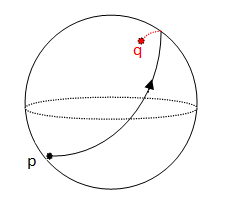# Lagrangian method for Christoffel symbols and Geodesics equations calculations

### Basic Concepts and principles

The Christoffel symbols calculations can be quite complicated, for example for dimension 2 which is the number of symbols that has a surface, there are 2 x 2 x 2 = 8 symbols and using the symmetry would be 6.

For dimension 4 the number of symbols is 64, and using symmetry this number is only reduced to 40. Certainly there are many calculations and this is just to find the equations of geodesics (after, we must solve or analyze it).

We will see in this section, the Lagrangian method allows us to obtain the geodesic equations and hence obtain the Chistoffel symbols. in a simpler way.

Ideas are the basis of the calculus of variations called principle of least action of Euler-Lagrange

First of all, a notation matter: from now on we will use the dot character "." to denote the derivate, this is a notation very used in physics, for example to denote $\frac{dx}{d\lambda}$ we will use $\dot{x}$.

### Principle of least action from Euler-Lagrange

Let $L\in{C^2(\mathbb{R}^3)}$ and let C be the set of functions

$y \in C^1([a,b])$ such us

y(a)=c, e y(b)=d if

$\int_a^b L(y(x), y'(x), x) dx$

reaches its minimum at some $y_0\in{C}$ then, $y_0$ is a solution of differential equation

$\frac{d}{dx}(\frac{{\partial L}}{{\partial y^{\prime}}}) - \frac{{\partial L}}{{\partial y}} = 0$

### Extended theory

All is based on the following Theorem

### Theorem

Let M be a m-dimensional variety and lets gij the metric tensor components. Then given a geodesic in M

$\gamma(\lambda) = (x^1(\lambda), ..., x^m(\lambda))$

Let L be the Lagrangian associated to the metric

$L=g_{ij} x\dot{_{i}}\dot{x_{j}}$

Then Euler-Lagrange equations are satisfied

$\frac{d}{d\lambda}(\frac{{\partial L}}{{\partial \dot{x}^i}}) - \frac{{\partial L}}{{\partial x^i}} = 0$

### Example

Lets the usual induced metric on a sphere S2 (Fig 1.), ie

$ds^2 = d \theta^2 + sin^2 \theta d\phi^2$Fig. 1 The sphere S2

We write the Lagrangian associated with this metric, ie

$L = \dot{\theta}^2 + sin^2 \theta \dot{\phi}^2$

And then, we calculate

$\frac{d}{d\lambda}(\frac{{\partial L}}{{\partial \dot{\theta}}}) = 2\ddot{\theta}$, $\frac{{\partial L}}{{\partial \theta}} = 2\dot{\phi}^2 sin \theta cos \theta$

$\frac{d}{d\lambda}(\frac{{\partial L}}{{\partial \dot{\phi}}}) = 2\ddot{\phi} sin^2 \theta + 4 \dot{\theta}\dot{\phi} sin \theta cos \theta$, $\frac{{\partial L}}{{\partial \ \phi}} = 0$

This allows us and write the equations of the geodesics, we obtain

$\left\{\begin{matrix} \ddot{\theta}- sin \theta cos \theta \dot{\phi}^2 = 0 \\ \ddot{\phi} + 2 \frac{cos \theta}{sin \theta} \dot{\theta} \dot{\phi} = 0 \end{matrix}\right.$

Remember that geodesic equations are

$\frac{d^2x^k}{d\lambda^2} + \Gamma_{ij}^k \frac{dx^i}{d\lambda}\frac{dx^j}{d\lambda} = 0$

We obtain the Chistoffel symbols too, are

$\Gamma_{22}^{1}=-sin\theta cos\theta$,

$\Gamma_{12}^{2}=\Gamma_{21}^{2}=\frac{cos\theta}{sin\theta}$

The rest of Christoffel symbols are zero.

# Was useful? want add anything?

Post here

### Carlos:

2015-03-24 13:00:00
Thanks marciliofds.

Well Theorem is consequence o of Euler-Lagrange Principle wich it is a classic Theorem in Calculus of variations (perhaps write in other form) you would find it on any book on this matter.

You can deduce the Theorem easily because if calculates derivates

$\frac{\partial L}{\partial \dot{x}^k}=2g_{kj} \dot{x}^i\dot{x}^j$

Then

$\frac{d}{d\lambda}( \frac{ \partial L}{ \partial \dot{x}^k})=2g_{kj,i}\dot{x}^i\dot{x}^j+2g_{kj}\ddot{x}^j$

And

$\frac{ \partial L}{ \partial x^k}=g_{ij,k}\dot{x}^i\dot{x}^j$

Now you can operate to calculate the Euler/Lagrange equations, adn deduce from it the geodesics equations (by contracting with metric contravariant Tensor $g^{lk}$. Thanks.
Carlos

### marciliofds:

2015-03-23 13:38:56
Where can i meet the theorem? Do you know a book?

Post here